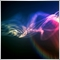# making a loop with bar154

Hi

I would like that each time there is a new bar formed,

my EA checks some instructions.

So I wrote that:

```count1 = iBars(NULL,PERIOD_D1);
count2 = count1;

while (etape == 0)
{
count1 = iBars(NULL,PERIOD_D1);
if(count2 =! count1)
{
if (cond1)
{
etape =1; // we move to the next step
}
else
{
count2= count2+1;
}
}
}      ```

But I keep wondering if should not have used a loop "FOR".

I thought of something like that:

```for(count=0; count<10; count++)
{
instructions;
}```

But the problem with the loop FOR, how could I make sure that each loop correspond to 1 day.

So that every instruction is only check when a new bar is formed?

Ludivine1055

I would like that each time there is a new bar formed,

my EA checks some instructions.

You could use a static to avoid the loop. This works with repeated calls to OnTick(). Like this:

```static int barcount=-1;
int count=iBars(NULL,PERIOD_D1);

if(count!=barcount && barcount!=-1) OnNewBarD1();
barcount=count;```154

lippmaje:

You could use a static to avoid the loop. This works with repeated calls to OnTick(). Like this:

I don't understand what is "OnNewBarD1();"

It is not a function of mql4.. so i am a bit confused1055

I don't understand what is "OnNewBarD1();"

It is not a function of mql4.. so i am a bit confused

It's a stub/placeholder for you to fill in.19099

Luciole:

`if(count!=barcount && barcount!=-1) NewBar = true;`